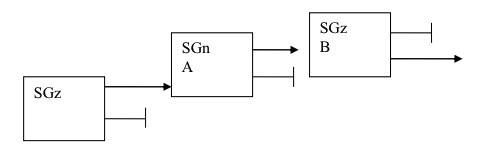# Represent Action of Stern Gerlach Operator as a Matrix

## Homework Statement

Given the series of three Stern-Gerlach devices:Represent the action of the last two SG devices as matrices ##\hat{A}## and ##\hat{B}## in the ##|+z\rangle, |-z\rangle## basis.

## Homework Equations

##|+n\rangle = cos(\frac{\theta}{2})|+z\rangle + e^{i\phi}sin(\frac{\theta}{2})|-z\rangle##

## The Attempt at a Solution

A Stern-Gerlach device with one beam blocked off can be represented as a projection of the incoming state onto the outgoing state. A projection operator can be written as the outer product of the selected basis state with itself, e.g. ##|+z\rangle\langle\,+\,z\,|## would be the projection operator for an SGz device with the minus size blocked.

The projection matrix of the first SGn is then:

##
\hat{A}\rightarrow{|+n\rangle\langle\,+\,n\,|} =
\begin{pmatrix}
cos(\frac{\theta}{2}) \\
e^{i\phi}sin(\frac{\theta}{2})
\end{pmatrix}
\begin{pmatrix}
cos(\frac{\theta}{2}) & e^{-i\phi}sin(\frac{\theta}{2})
\end{pmatrix} =
\begin{pmatrix}
cos^{2}(\frac{\theta}{2}) & e^{-i\phi}sin(\frac{\theta}{2})cos(\frac{\theta}{2}) \\
e^{i\phi}sin(\frac{\theta}{2})cos(\frac{\theta}{2}) & sin^{2}(\frac{\theta}{2})
\end{pmatrix}
##

Therefore, the beam coming out of the positive side of the SGn should be:

##
|+n\rangle = \hat{A}|+z\rangle \rightarrow
\begin{pmatrix}
cos^{2}(\frac{\theta}{2}) & e^{-i\phi}sin(\frac{\theta}{2})cos(\frac{\theta}{2}) \\
e^{i\phi}sin(\frac{\theta}{2})cos(\frac{\theta}{2}) & sin^{2}(\frac{\theta}{2})
\end{pmatrix}
\begin{pmatrix}
1 \\
0
\end{pmatrix} =
\begin{pmatrix}
cos^{2}(\frac{\theta}{2}) \\
e^{i\phi}sin(\frac{\theta}{2})cos(\frac{\theta}{2})
\end{pmatrix}
##

Should this be equal to the given ##|+n\rangle##?

I feel as though I'm missing something completely obvious or misunderstanding the question.

TSny
Homework Helper
Gold Member
Therefore, the beam coming out of the positive side of the SGn should be:

##
|+n\rangle = \hat{A}|+z\rangle \rightarrow
\begin{pmatrix}
cos^{2}(\frac{\theta}{2}) & e^{-i\phi}sin(\frac{\theta}{2})cos(\frac{\theta}{2}) \\
e^{i\phi}sin(\frac{\theta}{2})cos(\frac{\theta}{2}) & sin^{2}(\frac{\theta}{2})
\end{pmatrix}
\begin{pmatrix}
1 \\
0
\end{pmatrix} =
\begin{pmatrix}
cos^{2}(\frac{\theta}{2}) \\
e^{i\phi}sin(\frac{\theta}{2})cos(\frac{\theta}{2})
\end{pmatrix}
##

Should this be equal to the given ##|+n\rangle##?

I feel as though I'm missing something completely obvious or misunderstanding the question.

Hello and welcome to PF!
Yes, the output of SGn should be |+n>, up to a normalization factor.

So would ##\frac{1}{cos(\frac{\theta}{2})}## make for a suitable normalization factor? Is there a more formal way of determining this normalization factor?

TSny
Homework Helper
Gold Member
So would ##\frac{1}{cos(\frac{\theta}{2})}## make for a suitable normalization factor?
Yes
Is there a more formal way of determining this normalization factor?
Not that I can think of at the moment. The usual way to find the normalization factor for a state |ψ>is to just look at <ψ|ψ>. But, in your example, you can spot the normalization factor by inspection.

Yes

Not that I can think of at the moment. The usual way to find the normalization factor for a state |ψ>is to just look at <ψ|ψ>. But, in your example, you can spot the normalization factor by inspection.
Thanks for you help. I still don't see how ##\langle\,+\,n\,|+n\rangle## would give me the normalization factor though. I'm getting ##cos^{2}(\frac{\theta}{2}) + sin^{2}(\frac{\theta}{2}) = 1## which is what is expected.

TSny
Homework Helper
Gold Member
Let |ψ> be the output of GSn.

Ahh I see and I set this equal to 1. Thank you for your help!

Let |ψ> be the output of GSn.
This didn't work. I got a factor of ##\frac{1}{cos^{2}(\frac{\theta}{2})}## when evaluating ##\langle\psi|\psi\rangle## with ##|\psi\rangle## being the output of SGn.

##
\begin{pmatrix}
cos^{2}(\frac{\theta}{2}) &
e^{-i\phi}sin(\frac{\theta}{2})cos(\frac{\theta}{2})
\end{pmatrix}
\begin{pmatrix}
cos^{2}(\frac{\theta}{2}) \\
e^{i\phi}sin(\frac{\theta}{2})cos(\frac{\theta}{2})
\end{pmatrix} =
cos^{2}(\frac{\theta}{2})[cos^{2}(\frac{\theta}{2}) + sin^{2}(\frac{\theta}{2})] = cos^{2}(\frac{\theta}{2})
##

Last edited:
mfb
Mentor
The normalization factor gets applied to both occurences of ψ, so you need the square root of ##\langle\psi|\psi\rangle## as normalization factor.

The normalization factor gets applied to both occurences of ψ, so you need the square root of ##\langle\psi|\psi\rangle## as normalization factor.
Thank you. How does this then relate back to the matrix representation? Would it just be ##\frac{1}{cos(\frac{\theta}{2})}\begin{pmatrix}cos^{2}(\frac{\theta}{2}) & e^{-i\phi}sin(\frac{\theta}{2})cos(\frac{\theta}{2}) \\ e^{i\phi}sin(\frac{\theta}{2})cos(\frac{\theta}{2}) & sin^{2}(\frac{\theta}{2})\end{pmatrix}##

mfb
Mentor
I don't think it makes sense to normalize the matrix. The norm smaller than 1 indicates that some particles get lost, which has a physical meaning.